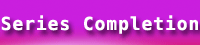# Series Completion Model Question Papers | Verbal Reasoning## Series Completion Sample Question

In each of the following series some of the letter(s) are missing which are given in that order as one of the alternatives below it. Choose the correct alternative.

1. ELFA, GLHA, ILJA, ?, MLNA
(a) OLPA
(b) KLMA
(c) LLMA
(d) KLLA

2. FGHJKLNOPRS?
(a) T
(b) M
(c) Q
(d) U

3. XGH, WIJ, VKL, UMN, ?
(a) TOP
(b) SOP
(c) HOW
(d) UJI

4. SCD, TEF, UGH, ?, WKL
(a) VLJ
(b) VJI
(c) CMN
(d) UJI

5. C, A, G, E, K, I, O, M, ?, ?
(a) US
(b) QS
(c) OQ
(d) SQ

6. LXF, MTJ, NPN, OLR, ?
(a) PIU
(b) PPV
(c) PHV
(d) PJW

7. BDF, CFI, DHL, ?
(a) EJO
(b) CJM
(c) EML
(d) EMI

8. ALN, DNR, GPR, ?
(a) KLM
(b) JRT
(c) RNU
(d) RNV

9. QPO, NML, KJI, ?, EDC
(a) HGF
(b) CAB
(c) JKL
(d) GHI

10. QAR, RAS, SAT, TAU, ?
(a) UAV
(b) UAT
(c) TAS
(d) TAT

11. GON, JRQ, MUT, ?
(a) NOG
(b) PXW
(c) JQR
(d) TUM

12. ACDFGIJLMOP?
(a) Q
(b) R
(c) T
(d) S

13. SFJ, RGK, QHL, ?
(a) EMD
(b) VIJ
(c) PIM
(d) PKL

14. a, k, e, o, i, s, ?, ?, q, a, u, e
(a) l, v
(b) m, v
(c) l, w
(d) m, w

15. KEC, LFD, MGE, NHF, ?
(a) OIF
(b) OIG
(c) PHG
(d) NIG

16. ABDC, GHJI, MNPO, ?
(a) RSQP
(b) STRO
(c) RSUT
(d) STVU

17. ACE, BDF, CEG, ?
(a) CED
(b) DFH
(c) DEM
(d) HED

18. AZBY, CXDW, EVFU, ?
(a) SHTG
(b) GXHW
(c) GTHS
(d) STHO

19. DKM, FJP, HIS, JHV, ?
(a) HGY
(b) LGZ
(c) IGY
(d) LGY

20. AZBY, ?, EVFU, GTHS
(a) BYCZ
(b) CYDR
(c) MISG
(d) CXDW